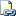# TRY_CONVERT (Transact-SQL)

Returns a value cast to the specified data type if the cast succeeds; otherwise, returns null.Transact-SQL Syntax Conventions

## Syntax

``````TRY_CONVERT ( data_type [ ( length ) ], expression [, style ] )
``````

## Arguments

• data_type [ ( length ) ]
The data type into which to cast expression.

• expression
The value to be cast.

• style
Optional integer expression that specifies how the TRY_CONVERT function is to translate expression.

style accepts the same values as the style parameter of the CONVERT function. For more information, see CAST and CONVERT (Transact-SQL).

The range of acceptable values is determined by the value of data_type. If style is null, then TRY_CONVERT returns null.

## Return Types

Returns a value cast to the specified data type if the cast succeeds; otherwise, returns null.

## Remarks

TRY_CONVERT takes the value passed to it and tries to convert it to the specified data_type. If the cast succeeds, TRY_CONVERT returns the value as the specified data_type; if an error occurs, null is returned. However if you request a conversion that is explicitly not permitted, then TRY_CONVERT fails with an error.

TRY_CONVERT is a new keyword in compatibility level 110.

This function is capable of being remoted to servers that have a version of SQL Server 2012 and above. It will not be remoted to servers that have a version below SQL Server 2012.

## Examples

### A. TRY_CONVERT returns null

The following example demonstrates that TRY_CONVERT returns null when the cast fails.

``````SELECT
CASE WHEN TRY_CONVERT(float, 'test') IS NULL
THEN 'Cast failed'
ELSE 'Cast succeeded'
END AS Result;
GO
``````

Here is the result set.

``````Result
------------
Cast failed

(1 row(s) affected)
``````

The following example demonstrates that the expression must be in the expected format.

``````SET DATEFORMAT dmy;
SELECT TRY_CONVERT(datetime2, '12/31/2010') AS Result;
GO
``````

Here is the result set.

``````Result
----------------------
NULL

(1 row(s) affected)
``````

### B. TRY_CONVERT fails with an error

The following example demonstrates that TRY_CONVERT returns an error when the cast is explicitly not permitted.

``````SELECT TRY_CONVERT(xml, 4) AS Result;
GO
``````

The result of this statement is an error, because an integer cannot be cast into an xml data type.

``````Explicit conversion from data type int to xml is not allowed.
``````

### C. TRY_CONVERT succeeds

This example demonstrates that the expression must be in the expected format.

``````SET DATEFORMAT mdy;
SELECT TRY_CONVERT(datetime2, '12/31/2010') AS Result;
GO
``````

Here is the result set.

``````Result
----------------------------------
2010-12-31 00:00:00.0000000

(1 row(s) affected)
``````